### Bisected Angle

Illustration to show that if an angle is bisected, and if a line drawn through the vertex perpendicular…### Bisected Angles

Illustration to show that the bisectors of two supplementary adjacent angles are perpendicular to each…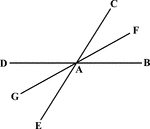### Bisected Angle

Illustration to show that the bisector of one of two vertical angles bisects the other.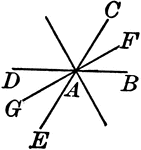### Bisected Angles

Illustration to show that the bisector of two pairs of vertical angles formed by two intersecting lines…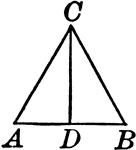### Bisected Vertical Angle of an Isosceles Triangle

Illustration to show that the bisector of the vertical angle of an isosceles triangle bisects the base,…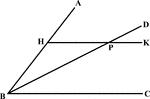### Angle Bisector Drawn Parallel to A Side

Illustration to show that if through any point in the bisector of an angle a line is drawn parallel…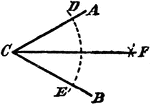### Construction Of A Divided Angle

An illustration showing the construction used to divide an angle into two equal parts. "With C as a…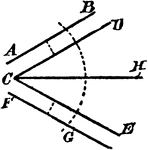### Construction Of A Divided Angle

An illustration showing the construction used to divide an angle into two equal parts when the lines…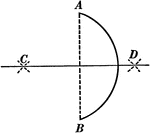### Bisecting an Arc

Illustration used to show how to bisect a given arc.### Circle With Diameter and Chord

Illustration of a circle with diameter and chord drawn. A diameter perpendicular to a chord bisects…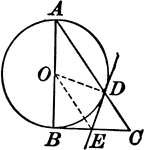### Circle With a Right Triangle

Illustration where one leg of a right triangle is the diameter of a circle. The tangent at the point…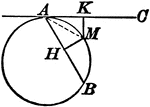### Circle With a Tangent Line and Chord

Illustration showing that from any point in the circumference of a circle, a chord and a tangent are…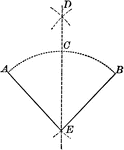### Construction of Bisecting a Given Angle

Illustration of the construction used to bisect a given angle.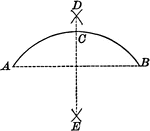### Construction of Bisecting a Given Arc

Illustration of the construction used to bisect a given arc.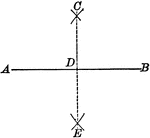### Construction of Bisecting a Given Line

Illustration of the construction used to bisect a given line.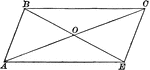### Parallelogram With Diagonals Bisecting Each Other

Illustration of a parallelogram with diagonals drawn to show they bisect each other.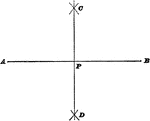### Bisect A Straight Line

Illustration used to show how to bisect a straight line.### Bisector of an Exterior Angle of an Isosceles Triangle

Illustration to show that the bisector of an exterior angle of an isosceles triangle, formed by producing…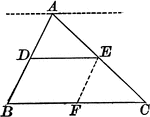### Triangle With Bisector Parallel to the Base

Illustration of a triangle showing that when a line parallel to the base of a triangle bisects one side,…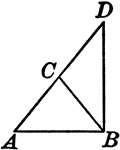### Leg Produced through the Vertex of an Isosceles Triangle

Illustration to show that if one of the legs of an isosceles triangle is produced through the vertex…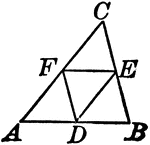### Midpoints of Triangle Divide Triangle into Four Equal Triangles

Illustration to show that if the lines joining the middle points of the sides of a triangle divide the…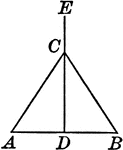### Perpendicular Bisector of the Base of an Isosceles Triangle

Illustration to show that the perpendicular bisector of the base of an isosceles triangle passes through…